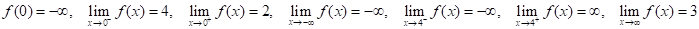Chapter 2.6, Problem 9E### Single Variable Calculus: Early Tr...

8th Edition
James Stewart
ISBN: 9781305270343

#### Solutions

Chapter
Section### Single Variable Calculus: Early Tr...

8th Edition
James Stewart
ISBN: 9781305270343
Textbook Problem

# Sketch the graph of an example of a function f that satisfies all of the given conditions.To determine

To sketch: The graph of f(x) which satisfies the conditions, f(0)=3,limx0f(x)=4, limx0+f(x)=2, limxf(x)=, limx4f(x)=, limx4+f(x)= and limxf(x)=3.

Explanation

Recall the definition of horizontal asymptote, “the line y=L is called a horizontal asymptote of the curve y=f(x) if limxf(x)=L or limxf(x)=L”.

Recall the definition that the line x=a is said to be a vertical asymptote of the function f(x), if at least one of the following conditions must be true: limxaf(x)=±, limxa+f(x)=±, limxaf(x)= and limxaf(x)=.

The equation limxf(x)= means that the graph of the curve approaches negative infinity as x approaches negative infinity.

The graph must contain a point (0, 3) as f(0)=3. So, mark the point (0, 3) as a filled dot.

The equation limx0f(x)=4 means that the graph of the curve approaches 4 but x4 as x approaches 0 from left

### Still sussing out bartleby?

Check out a sample textbook solution.

See a sample solution

#### The Solution to Your Study Problems

Bartleby provides explanations to thousands of textbook problems written by our experts, many with advanced degrees!

Get Started

#### Find more solutions based on key concepts